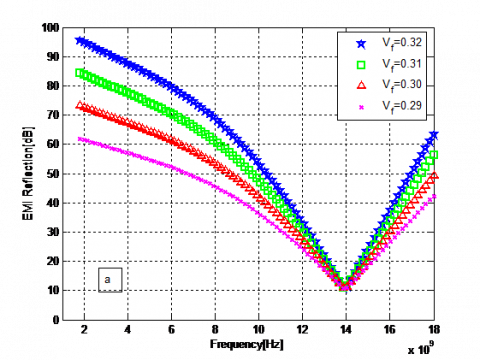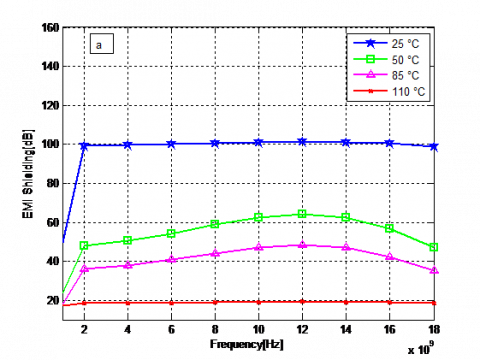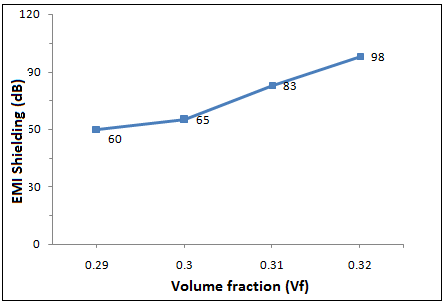# Impact of Temperature Variation on the Electromagnetic Shielding Behavior of Multilayer Shield for EMC Applications

Impact of Temperature Variation on the Electromagnetic Shielding Behavior of Multilayer Shield for EMC Applications
Semiconductors and Functional Materials Laboratory, Faculty of Technology, Department of Electronics, University of Laghouat B.P. 37G, Laghouat 03000, Algeria

Laboratory for Analysis and Control of Energy Systems and Electrical Systems LACoSERE, Faculty of Technology, Department of Electronics, University of Laghouat B.P. 37G, Laghouat 03000, Algeria

Corresponding Author Email:
t.merizgui@lagh-univ.dz
Page:
363-367
|
DOI:
https://doi.org/10.18280/rcma.290604
26 August 2019
|
Accepted:
15 October 2019
|
Published:
23 December 2019
| Citation

OPEN ACCESS

Abstract:

This paper attempts to disclose how temperature variation affects the electromagnetic shielding of a multilayer composite made of copper (Cu) fibers surrounded by silicon (Si). The model of the composite was fabricated by physical vapor deposition method, and tested with the temperature rising from room temperature (RT), 50ºC, 85ºC to 110ºC. Under these temperatures, several electromagnetic shielding parameters of the composite were measured in various operating microwave frequencies (E, F, I and J bands). Among them, dielectric permittivity was acquired by LCR tester, magnetic permeability was measured by vibrating sample magnetometer (VSM), and the electromagnetic (EM) wave attenuation was recorded by typical microwave test bench. The results show that the composite had an EMI shielding of 20dB at 85º and 100dB at the RT; the reflection loss of the composite (volume fraction: 0.32) was also 100dB at the RT. Thus, the temperature rise suppresses the reflection loss; the composite has better electromagnetic shielding effect at the RT than at elevated temperatures; the volume faction enhances the wave attenuation of the composite. The results provide a good reference for the design of stable electromagnetic shielding materials at elevated temperatures.

Keywords:

electromagnetic compatibility EMC, materials, temperature, electrical conductivity

1. Introduction

This paper aims for better understanding the relations between the parameters such as electrical conductivity, shielding effectiveness mechanisms and the temperature effect of the shielding material against the electromagnetic interference (EMI). Here, novel multilayer composites made of copper (Cu) fibres surrounded by silicon (Si) which are of particular interest. The insulating layer (Si) could be sandwiched between two composites of (Cu fibre) in same thickness with perfect electrical conductivity, which resulting a better electromagnetic shielding performance. A novel multi-layer structure, which could be made of two-layered composite (conductive) separated by a dielectric layer (0.25 µm thick) to see how the multi-layered composite structure works out. The structure has the same composite layer containing 50 copper fibres with an σ = 5.8*107 of S/m, and 0.036 µm radius embedded inside the dielectric layer (Si) ɛ = 11.9 with 0.5 µm thick. This multilayer structure (Copper fibre/Si) could increase the shielding effectiveness (SE) by increasing the multiple internal reflections waves between the dielectric layers which has a direct positive effect on the total shielding effectiveness.

2. Experimental Procedure

2.1 EMI shielding model

The SE of a material is defined by the ratio of the transmitted power (Pt) through the material to the incident power (Pi) of an electromagnetic wave. In this paper, we have proposed a new multi-layer structure with two conductive layers (1 and 3) separated by a dielectric layer (Si) as shown in Figure 1.

## 1.pngFigure 1. Proposed multi layer structure CuF/Si-Si-CuF/Si

When the wave impinges the slab, a part of wave energy reflected and the other absorbed or transmitted, the scattering parameters (S-parameters) can be used to define the quality of a shield

A=1-R-T.                                 (1)

where, R and T are the reflection and transmission power respectively, calculated based on the S parameters; it can be defined from the VNA as follows :

$R={{\left| {{S}_{11}} \right|}^{2}}={{\left| {{S}_{22}} \right|}^{2}},T={{\left| {{S}_{12}} \right|}^{2}}={{\left| {{S}_{21}} \right|}^{2}}$                (2)

And

${{S}_{11}}={{S}_{22}}=\frac{\Gamma \left( 1-{{T}^{2}} \right)}{1-{{\Gamma }^{2}}{{T}^{2}}},{{S}_{21}}={{S}_{12}}=\frac{T\left( 1-{{\Gamma }^{2}} \right)}{1-{{\Gamma }^{2}}{{T}^{2}}}$           (3)

where, |Sij|2 represents the power reflection (i=j) and transmission (ij) from port i to port j. So the total resistance of the composite can be obtained as [10-13]:

$\frac{1}{{{R}_{C}}}=\frac{1}{{{R}_{f1}}}+\frac{1}{{{R}_{f2}}}+....+\frac{1}{{{R}_{f58}}}+\frac{1}{{{R}_{m}}}$                  (4)

When considering the expression $R=\frac{L}{A}\times \frac{1}{\sigma }$in Eq. (4), we got after some manipulation:

${{\sigma }_{C}}={{\sigma }_{f}}\sum{\frac{{{A}_{fi}}}{{{A}_{C}}}+{{\sigma }_{m}}\frac{{{A}_{m}}}{{{A}_{C}}}}$                       (5)

${{\sigma }_{C}}={{\sigma }_{f}}{{V}_{f}}+{{\sigma }_{m}}(1-{{V}_{f}})$                    (6)

In the situation of an insulating matrix, in which $\sigma \approx 0$, Eq.8 minimize to:

${{\sigma }_{C}}\approx {{\sigma }_{f}}\times {{V}_{f}}$                               (7)

where, σc is electrical conductivity of the composite (S/m), σm is electrical conductivity of the matrix (S/m), Vf is volume fraction of the copper fibres. Rm, Rc, Rf, represent the resistance of the matrix, composite and fiber respectively, A is cross-section area of fuselage skin (m2). Mostly, fiber volume fraction is calculated according to ASTM D2584 as

${{V}_{f}}=\left[ {{\rho }_{m}}\times {{w}_{f}}/\left( {{\rho }_{m}}\times {{w}_{f}}+{{\rho }_{f}}\times {{w}_{m}} \right) \right]$           (8)

where, Vf volume fraction of fibers, Wf weight of fibers, Wm weight of matrix, ρf density of fibers, ρm density of matrix.

2.2 Shielding material preparation

The multilayer composite material was prepared and analyzed theoretically by ‘HFSS software, high-frequency structure simulator’ process and the real time model was prepared using PVD process. In that the substrate material used was copper fibres of 100 nos arranged as systematic format. The silica nano material was forced to deposit on the material in vacuum environment. The layer thickness achieved here was 0.5 µm in both side of copper fibre. The substrate temperature was cooled to room temperature by room temperature cooling and inspected for visual defects.

2.3 Testing of composites

The electrical conductivity and insulation behavior were analyzed using an LCR meter, HIOKI, JAPAN. The EMI shielding effect of fabricated multilayer composite was analyzed using microwave test bench attached with Klystron setup for microwave production. The sample was placed in a movable shot and reflection wave attenuation was measured using a VSWR meter in terms of dB. The test was repeated several times to find average. Figure 2 shows the typical microwave test bench used for the current study.

## 2.pngFigure 2. Microwave test bench setup

3. Results and Discussion

3.1 The effect of temperature on the proposed multilayer composite

Figure 3(a) displayed the variation of electrical conductivity vs volume fraction, and Figure 3(b) presents the temperature depending on the electrical conductivity (S/m) of silicon matrix, and electrical conductivity (S/m) of fibers (Cu) as a function of 1000/T. The acquired curves decrease linearly when the temperature increase, according to the Arrhenius law :

${{\sigma }_{dc}}T={{A}_{0}}\exp -\left( \frac{{{E}_{a}}}{{{k}_{B}}T} \right)$                          (9)

where, A0 is the pre-exponential factor, Ea is the activation energy, and kB is the Boltzmann constant.

It is observed that when volume fraction of particle increases the conductivity get increases. This increment is because of improved conductivity via inclusion of conductive particles . Both volume fraction and conductivity are directly proportional based on the curve in Figure 3(a). The Figure 3 shows the temperature effect on conductivity. When temperature increases in all volume fractions same conductivity is observed. It is because of increased temperature affected the conductivity of composite material by increasing the kinetic energy of particles . The Table 1 shows the electrical conductivity obtained from the proposed sample.

## 3.pngFigure 3. Variation in electrical conductivity vs volume fraction and temperature dependence of Ln (σT) for proposed composite

Table 1. Electrical conductivity obtained from the proposed sample

 Sample proposed σ(S/m) at T = 25 °C σ(S/m) at T = 50 °C σ(S/m) at T=85 °C σ(S/m) at T= 110 °C Ref. Copper (CuF) 5.89*107 5 *107 4.119*107 3.656*107 Our work Silicon (Si) 0.896*10-3 0.73*10-3 0.601*10-3 0.472*10-3 Our work

3.2 The effect of temperature on the electromagnetic characteristics

The effect of temperature on the electrical conductivity of Copper (CuF) fibre, silicon matrix, and the electrical parameters responses of the whole proposed composite sample are presented in Figures 4 and 5. It is noted that when frequency increases from 2 GHz to 8 GHz the reflection losses were decreased and saturated at 10 dB. Whereas when the frequency again increases to 10 GHz the reflection loss further increases up to 80 dB. The volume fraction of copper in multilayer composite was 0.32. This reduction in higher frequency is the cause of increased frequency, which penetrates the shielding material at faster rate. The amount energy reduction within the skin depth is very less due to higher amplitude . Thus reduction in reflection loss was observed. It is observed that in Figure 4(b) the wave reflection loss suddenly increases with increase of temperature. The reflection loss of 87 dB was observed in room temperature for shielding material having 0.32 volume fractions. But when temperature reaches 85ºC the reflection loss was reduced to 14dB. Thus reduction is the cause of poor conductivity of composite layer under elevated temperature. In higher temperature the molecules got activation energy and hence they start rotating. Thus poor polarization occurs and reduced the charge flow. The following simulation results of the reflection and the shielding effectiveness have proved the efficiency of the analytical model that used.

## 4a.png(a) EMI reflection vs volume fraction and frequency at 25 °C

## 4b.png(b) EMI reflection vs temperature and frequency at Vf=0.32

Figure 4. Simulation results of multilayer CuF/Si

## 5a.png(a) EMI SE vs temperature and frequency at Vf=0.32

## 5b.png(b) EMI SE vs volume fraction and frequency at 25 °C

Figure 5. Simulation results of multilayer CuF/Si

## 6.pngFigure 6. EMI shielding vs. volume fraction

Figure 4 and 5 shows the EMI reflection loss of various multilayer composites fabricated using different volume fraction of copper wire and silicon layer. It is noted that when volume fraction increases the shielding effect also increases. This increment is the cause of improved conductivity of multilayer composite. Figure 6 shows the relationship between EMI shielding and volume fraction of composites. The reflection loss of 40 dB was observed for composite contain 0.29 Vf whereas the improvement of 17%, 27% and 37.5% were observed when 0.3 Vf, 0.31 Vf and 0.32 Vf of second phase additions added into a multilayer composite. This improvement is the cause of presence of higher conductivity copper micro-wires. When incoming micro-wave hits the shielding material the eddy current created within the material and it hampered the electron flow further to pass over. This resistance in electron flow absorbs most of the electrical component of incoming micro-wave thereby attenuates the microwave

4. Conclusions

The following are the conclusions made from the current work. The obtained results from this work prove that the proposed arrangement built from alternating composite of (CuF/Si) and dielectric layers of silicon (Si) displays a better performance of SE by high reflection mechanism between the layers. Same time the temperature increase changes the reflection loss to lower level. In room temperature the composites show better SE properties than elevated temperatures. The increase in volume fraction increased the wave attenuation behavior of multi-layer composite. Therefore, in order to achieve the better shielding properties of composite materials the thermally insulative coating materials on shielding composites are to be applied to avoid temperature interruptions. These thermally non conductive shielding materials having the advantage of highly stable shielding effectiveness material in high temperature wave propagation application.

Conflict of Interest

Herewith authors have confirmed that, this article have no conflict of interests.

Nomenclature
 EMI Electromqgnetic interference SE Shielding effectiveness HFSS high-frequency structure simulator Greek ymbols σc Electrical conductivity of composite σf Electrical conductivity of fibre σc Electrical conductivity of matrix Subscripts T temperature A0 pre-exponential factor CuF Copper fiber
References

 Tahar, M., Abdechafik, H., Mecheri, K. (2018). Modelling and measurement of electromagnetic shielding effectiveness. International Conference on Electrical Sciences and Technologies in Maghreb (CISTEM). IEEE, pp. 1-6. https://doi.org/10.1109/CISTEM.2018.8613459

 Merizgui, T., Hadjadj, A., Kious, M., Gaoui, B. (2019). Effect of human body temperature on new multilayer composite shield in pacemaker. Revue des Composites et des Materiaux Avances, 29(1), 27-32. https://doi.org/10.18280/rcma.290105

 Merizgui, T., Hadjadj, A., Gaoui, B., Kious, M. (2018). Comparison electromagnetic shielding effectiveness between smart multilayer arrangement shields. 2018 International Conference on Applied Smart Systems (ICASS). IEEE, pp. 1-5. https://doi.org/10.1109/ICASS.2018.8651965

 Gaoui, B., Hadjadj, A., Kious, M. Novel multilayer arrangement of conductive layers traps the electromagnetic interferences by multiple internal reflections at high frequency in the far field. Journal of Materials Science: Materials in Electronics, 28(4): 3924-3930. https://doi.org/10.1007/s10854-016-6006-z

 Viswanathan, R., Arun praksh, V.R. (2018). Microwave shielding behavior of silanized Cu and Cu-Fe3O4 compound particles reinforced epoxy resin composite in E, F, I and J band frequencies. Polymer Bulletin, 75(9): 4207-25. http://dx.doi.org/10.1007/s00289-017-2262-1

 Vincent, A., Ramesh, G., Kumar, S.M. (2018). Microwave shielding behaviour of surface treated MWCNT-epoxy composites in I & J band-A note. Colloid and Interface Science Communications, 24: 89-92. https://doi.org/10.1016/j.colcom.2018.04.007

 Merizgui, T., Hadjadj, A. Kious, M., Gaoui, B. (2019). Enhanced of electrical properties and shielding efficiency of hybrid composite with temperature. Revue des Composites et des Matériaux Avancés, 29(3): 171-177. https://doi.org/10.18280/rcma.290307

 Arun prakash, V.R., Rajadurai, A. (2016). Radio frequency shielding behavior of silane treated Fe2O3/E-glass fibre reinforced epoxy hybrid composite. Applied Physics A., 122: 875-882. https://doi.org/10.1007/s00339-016-0411-2

 Joshi, A., Datar, S. (2015). Carbon nanostructure composite for electromagnetic interference shielding. Prmana, 84(6): 221-229.

 Merizgui, T., Hadjadj, A., Kious, M., Arun Prakash, V.R., Gaoui, B. (2018). Effect of magnetic iron (III) oxide particle addition with MWCNTs in kenaf fibre-reinforced epoxy composite shielding material in ‘E’, ‘F’, ‘I’ and ‘J’ band microwave frequencies. Materials Research Express, 6(4): 046102. https://doi.org/10.1088/2053-1591/aaf9de

 Ning, P. (1993). Theoretical determination of the optimal fiber volume fraction and fiber‐ matrix property compatibility of short fiber composites. Polymer composites, 14(2): 85-93. https://doi.org/10.1002/pc.750140202

 Gibson, B., Cumiskey, S., Christopher, W. (2002). Compaction of fiber reinforcements. Polymer composites, 23(3): 307-318. https://doi.org/10.1002/pc.10433

 Bozkurt, N., Yazicioğlu, S., Christopher, W. (2017). The strength properties of fibre reinforced self compacting concrete. Acta Physica Polonica, 132(3): 775-778.

 Merizgui, T., Hadjadj, A., Kious, M., Gaoui, B. (2019). Effects of temperature on the electromagnetic shielding effectiveness under a plane wave excitation. International Journal of Digital Signals and Smart Systems.

 Merizgui, T., Hadjadj, A., Gaoui, B., Kious, M. (2018). Effect of temperature on the electromagnetic characteristic behavior of copper. 2018 International Conference on Applied Smart Systems (ICASS), IEEE, pp, 1-5. https://doi.org/10.1109/ICASS.2018.8652063

 Hussian, W.A., Hussian, A.A. (2015). Dielectric properties and A.C conductivity of epoxy/alumina silicate NGK composites. Advances in Chemical Engineering and Science, 5: 282-289. https://doi.org/10.4236/aces.2015.53028